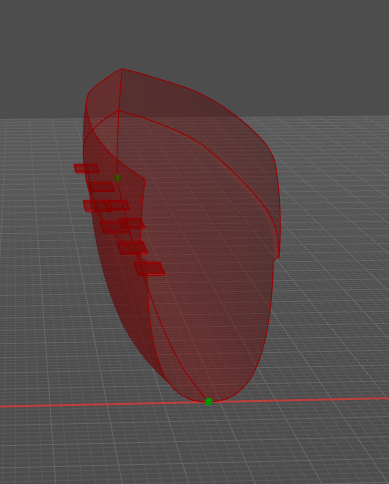# Failure with the mirror

hi, i’m having a trouble with a mirror. At first when I create it it’s alright (like on the first picture). But at some point, while working on the script, the mirror stops working. The guid of “mirrored object” stays, but it is no longer mirrored (in the second picture you can see that both surfaces overlap on the same place). Whenever I restart the program, it is either correct or not working again.

the line looks like this:

mirHull = rs.MirrorObject(loftHull, axPoStart, axPoEnd, True)

and it doesn’t change throughout the work process, nor objects used in it. No error returns.

is someone familiar with this problem? what could be wrong?P.S. also noticed that it occurs whenever I press test/ok from front view, but was alright from perspective. Is it bug?

You have basically answered your own question here. The RhinoScript function you’re using is dependent on the CPlane of the currently active Rhino viewport.

Here’s the code for the RhinoScript function you are calling:

``````def MirrorObject(object_id, start_point, end_point, copy=False):
"""Mirrors a single object
Parameters:
object_id (guid): The identifier of an object to mirror
start_point (point): start of the mirror plane
end_point (point): end of the mirror plane
copy (bool, optional): copy the object
Returns:
guid: Identifier of the mirrored object if successful
None: on error
Example:
import rhinoscriptsyntax as rs
obj = rs.GetObject("Select object to mirror")
if obj:
start = rs.GetPoint("Start of mirror plane")
end = rs.GetPoint("End of mirror plane")
if start and end:
rs.MirrorObject( obj, start, end, True )
MirrorObjects
"""
rc = MirrorObjects(object_id, start_point, end_point, copy)
if rc: return rc
``````

As you can see, it is just calling the function:
`MirrorObjects(object_id, start_point, end_point, copy)`
It’s code is here:

``````def MirrorObjects(object_ids, start_point, end_point, copy=False):
"""Mirrors a list of objects
Parameters:
object_ids ([guid, ...]): identifiers of objects to mirror
start_point (point): start of the mirror plane
end_point (point): end of the mirror plane
copy (bool, optional): copy the objects
Returns:
list(guid, ...): List of identifiers of the mirrored objects if successful
Example:
import rhinoscriptsyntax as rs
objs = rs.GetObjects("Select objects to mirror")
if objs:
start = rs.GetPoint("Start of mirror plane")
end = rs.GetPoint("End of mirror plane")
if start and end:
rs.MirrorObjects( objs, start, end, True )
MirrorObject
"""
start_point = rhutil.coerce3dpoint(start_point, True)
end_point = rhutil.coerce3dpoint(end_point, True)
vec = end_point-start_point
if vec.IsTiny(0): raise Exception("start and end points are too close to each other")
normal = scriptcontext.doc.Views.ActiveView.ActiveViewport.ConstructionPlane().Normal
vec = Rhino.Geometry.Vector3d.CrossProduct(vec, normal)
vec.Unitize()
xf = Rhino.Geometry.Transform.Mirror(start_point, vec)
rc = TransformObjects(object_ids, xf, copy)
return rc
``````

Pay attention to these lines:

``````    normal = scriptcontext.doc.Views.ActiveView.ActiveViewport.ConstructionPlane().Normal
vec = Rhino.Geometry.Vector3d.CrossProduct(vec, normal)
vec.Unitize()
xf = Rhino.Geometry.Transform.Mirror(start_point, vec)
``````

It is using the CPlane of the currently active Rhino viewport to calculate the normal vector of the mirror plane used in the transformation.

-Kevin

1 Like

is there another mirror command that will take into account 3d shape and not just one screen? this is just awkward. so if i have mirror somewhere in the beginning of my script and want to test something else that is better seen from a specific view - my whole geometry crumbles from that mirror now.